﻿ Simple Calculator in JavaFX

# Simple Calculator in JavaFX

## JavaFx Basic: Exercise-7 with Solution

Write a JavaFX application that creates a basic calculator application that can add, subtract, multiply, and divide two numbers entered by the user.

Sample Solution:

JavaFx Code:

``````import javafx.application.Application;
import javafx.geometry.Insets;
import javafx.scene.Scene;
import javafx.scene.control.Button;
import javafx.scene.control.Label;
import javafx.scene.control.TextField;
import javafx.scene.layout.GridPane;
import javafx.stage.Stage;

public class Main extends Application {

private TextField num1Field;
private TextField num2Field;
private Label resultLabel;

public static void main(String[] args) {
launch(args);
}

@Override
public void start(Stage primaryStage) {
primaryStage.setTitle("Basic Calculator");

GridPane grid = new GridPane();
grid.setHgap(10);
grid.setVgap(10);

num1Field = new TextField();
num1Field.setPromptText("Enter first number");
GridPane.setConstraints(num1Field, 0, 0);

num2Field = new TextField();
num2Field.setPromptText("Enter second number");
GridPane.setConstraints(num2Field, 0, 1);

resultLabel = new Label("Result: ");
GridPane.setConstraints(resultLabel, 0, 2);

Button subtractButton = new Button("Subtract");
subtractButton.setOnAction(e -> performOperation('-'));
GridPane.setConstraints(subtractButton, 2, 0);

Button multiplyButton = new Button("Multiply");
multiplyButton.setOnAction(e -> performOperation('*'));
GridPane.setConstraints(multiplyButton, 1, 1);

Button divideButton = new Button("Divide");
divideButton.setOnAction(e -> performOperation('/'));
GridPane.setConstraints(divideButton, 2, 1);

Scene scene = new Scene(grid, 300, 200);
primaryStage.setScene(scene);
primaryStage.show();
}

private void performOperation(char operator) {
String num1Text = num1Field.getText();
String num2Text = num2Field.getText();

if (isValidNumber(num1Text) && isValidNumber(num2Text)) {
double num1 = Double.parseDouble(num1Text);
double num2 = Double.parseDouble(num2Text);
double result = 0.0;

switch (operator) {
case '+':
result = num1 + num2;
break;
case '-':
result = num1 - num2;
break;
case '*':
result = num1 * num2;
break;
case '/':
if (num2 != 0) {
result = num1 / num2;
} else {
resultLabel.setText("Result: Error (Division by zero)");
return;
}
break;
default:
break;
}

resultLabel.setText("Result: " + result);
} else {
resultLabel.setText("Result: Invalid Input");
}
}

private boolean isValidNumber(String text) {
return text.matches("-?\\d*\\.?\\d+");
}
}
```
```

Explanation:

In the exercise above -

• Import the necessary JavaFX libraries.
• Create a class "Main" that extends "Application" and overrides the "start()" method.
• Inside the "start()" method:
• Set the application window title to "Basic Calculator."
• Create a GridPane for organizing UI elements with specific spacing and padding.
• Create two text fields (num1Field and num2Field) for users to enter the first and second numbers. Set placeholders for user guidance.
• Create a label (resultLabel) to display the calculation result.
• Create four buttons (addButton, subtractButton, multiplyButton, and divideButton) for addition, subtraction, multiplication, and division. Each button is configured to trigger a specific action when clicked.
• Use 'GridPane.setConstraints' to position these UI elements within the grid.
• Create a Scene with the grid layout, specifying the window dimensions as 300x200.
• Set the created scene in the stage (primaryStage) and show the window.
• The "performOperation()" method:
• Reads the user input from the text fields.
• Validates the input to ensure it's a valid number.
• Performs the selected operation (addition, subtraction, multiplication, or division) on the two numbers.
• Updates the 'resultLabel' with the calculated result or an error message in case of invalid input or division by zero.
• The "isValidNumber()" method checks whether the input text is a valid number using a regular expression.

Sample Output:

```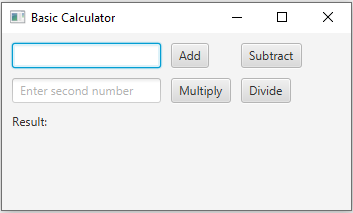```
```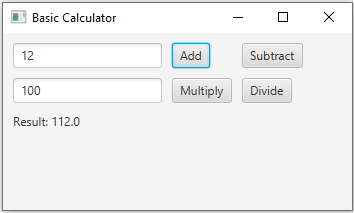```
```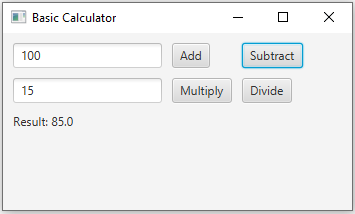```
```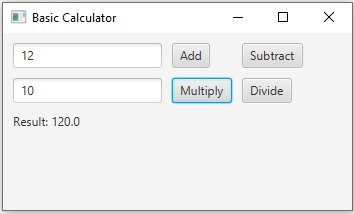```
```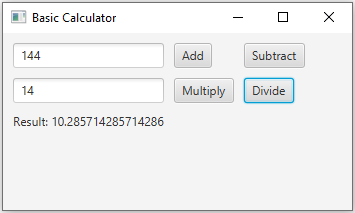```

Flowchart: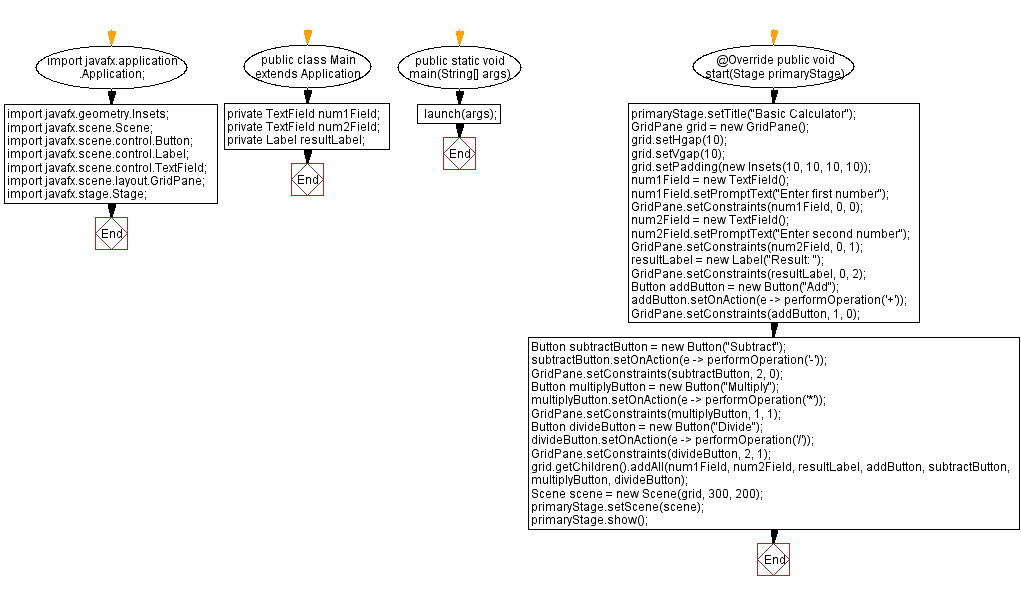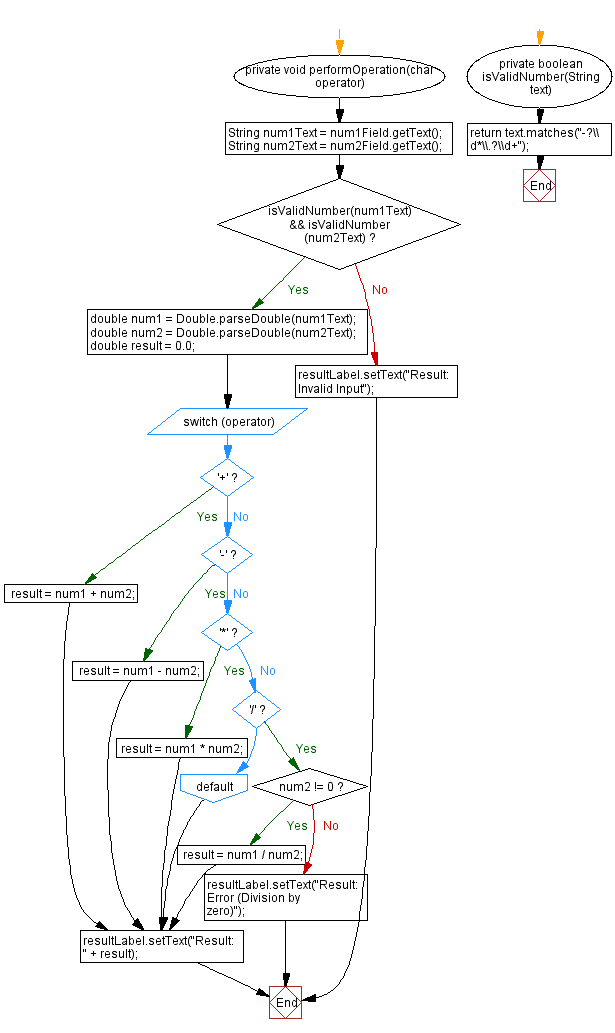Java Code Editor:

Previous:Image display in JavaFX.
Next: Styling with CSS in JavaFX.

What is the difficulty level of this exercise?

Test your Programming skills with w3resource's quiz.

﻿